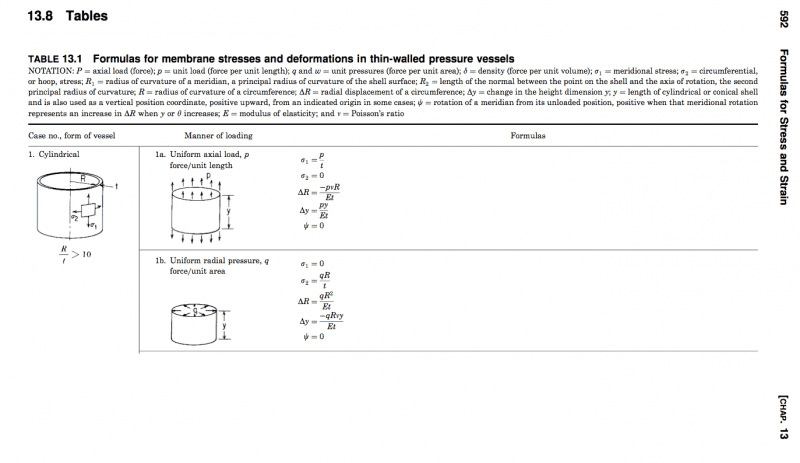# Large Diameter Interference Fit: Removal Load

Hello. I have 2 large diameter cylindrical shells that are "rabbetted" together. I am trying to determine the approximate load required to pull them apart without much success. The parameters are as follows (all length dimensions in inches) and result in a .005" (diametric) interference:

Outer Shell (female):
OD2 = 40.525
ID2 = 40.175
t2 = .350

Inner Shell (male):
OD1 = 40.180
ID1 = 39.98
t1 = .200

The axial engagement length of the fit is:
L = .125
The materials are the same for inner & outer shell:
v = 0.3
E = 221 GPa

I have tried a couple of approaches that yield very different numbers. I first tried just considering the force required to deflect the inner shell by the interference value (radially .0025") and then using this "normal force" to determine the frictional load to overcome. I used a formula in Roarks to determine this force. This requires a significant amount of force compared to using a "shaft/hub" press fit calculator such as the one at this tribology site. The difference in answers is from about 1200 lbf to 20 lbf, respectively.

I think a couple of things are happening here, but could use some guidance:

1) I believe that the Roarks approach might be overly conservative since it does not consider the outer shell and that it will expand a little to accommodate the inner shell.

2) For a shaft/hub equation, I am guessing that they might not be too accurate for "shafts/hubs" as large as the ones I am looking at. But maybe someone could comment on this?

Baluncore
There is a game changing strategy.

The easiest way to separate the two is to pull the near end of the inner shell while pushing the near end of the outer shell. That is because, when the inner shell is stretched, it's diameter will contract slightly, and when the outer shell is compressed it's diameter will expand slightly.

Pressing the inner shell through the outer shell supported at the near end will have the opposite effect and tend to lock them together.

There is a game changing strategy.

The easiest way to separate the two is to pull the near end of the inner shell while pushing the near end of the outer shell. That is because, when the inner shell is stretched, it's diameter will contract slightly, and when the outer shell is compressed it's diameter will expand slightly.

Pressing the inner shell through the outer shell supported at the near end will have the opposite effect and tend to lock them together.

Hi Baluncore :) I appreciate the thoughts. I do still, however, need to calculate the removal loads.

Baluncore
If the two methods had given consistent numbers, would you have believed the result?

Are you trying to find out how hard it will be to dismantle them later?
If so it will depend on how you hold them.

Or are you trying to make sure they will not come apart during use?
That will depend on how you use them.

As I said, I am trying to determine the removal load. We have an interference fit. Like a shaft & collar. When it's time to disassemble, we need to know what kind of load we are looking at, regardless of how it is held.

****NEW APPROACH****

Here is an approach I would like to to try. Assume that we have 2 "thin" cylinders of length L, inner radius R, and thickness t. Using thin shell theory we can relate the radial deflection of a cylinder to the uniform pressure acting on its internal or external face. See Table 13.1, Case 1b from Roark's Formulas for Stress and Strain below. These formulas basically assume that the whole shell deflects inward or outward and that the thickness does not change.

Let the subscript 1 denote the inner "male" cylinder and the subscript 2 the outer "female" cylinder.

We know the following about the final state of the cylinders when they are are fully "fitted":

1) The magnitude of the pressure acting at the interface is the same, just the sign is reversed.
2) The outer radii of the "male" part must be the same as the inner radii of the "female" part.
3) The change in radii of the male & female parts must sum to the interference value.

Let $R'$ denote the "final" value at equilibrium; the conditions from above can be written as;

$|q_1| = |q_2| = p \qquad(1)$

$R'_1 + t_1 = R'_2 \qquad(2)$

$\Delta R_1 + \Delta R_2 = \delta \qquad(3)$

Using the formula from case 1b in Table 13.1, taking the radially outward direction as positive, we can re-write equations (2) & (3) in terms of the initial known values as:

$R_1 -\frac{pR_1^2}{E_1t_1}+ t_1 = R_2 + \frac{pR_2^2}{E_2t_2} \qquad(2')$

$\frac{-pR_1^2}{E_1t_1} + \frac{pR_2^2}{E_2t_2}= \delta \qquad(3')$

My Problem is that I am having trouble with the math here. Somehow, equation 2' is solvable for "p" but is not constrained to values for which 3' holds. Is this doable analytically? Did I overlook something here in order to "couple" 2' & 3'? Or do I need an iterative approach to couple these?

EDIT: My first thought is to substitute 3' into 2' by using something like:

$\frac{pR_2^2}{E_2t_2}= \frac{pR_1^2}{E_1t_1} + \delta$

but for some reason I am hesitant....

Thanks for looking.Last edited: